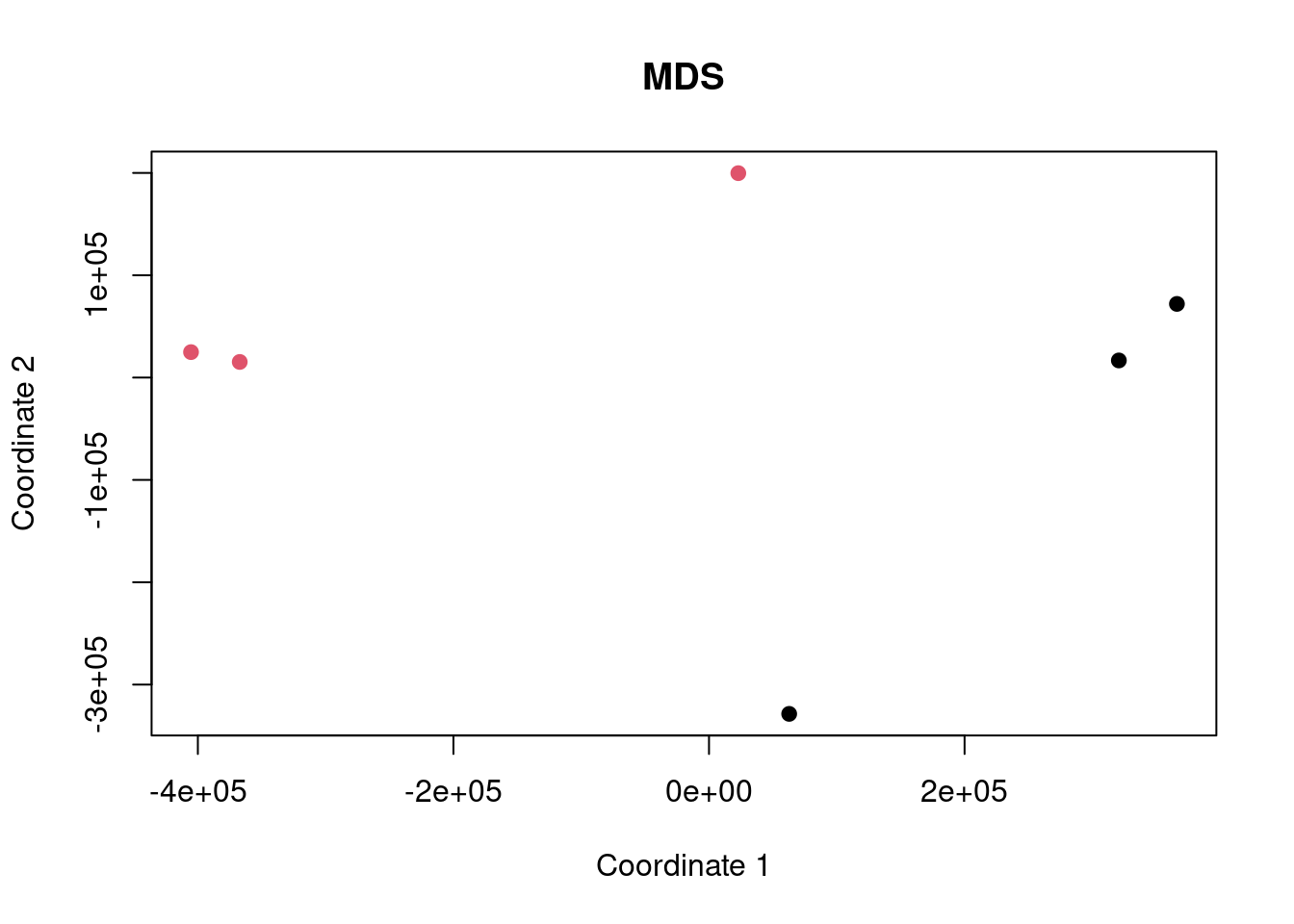## Intro

Here we are performing an analysis of some gene expression data to demonstrate the difference between ORA and FCS methods and to highlight the differences caused by improper background gene set use.

The dataset being used is SRP096178 and we are comparing the cells grown in normal condition (control) to those grown with addition of SAHA (case).

Data are obtained from http://dee2.io/

``````suppressPackageStartupMessages({
library("getDEE2")
library("DESeq2")
library("clusterProfiler")
library("mitch")
library("kableExtra")
library("eulerr")
})``````

## Get expression data

I’m using some RNA-seq data looking at the effect of SAHA on HAEC cells.

``````name="SRP037718"
samplesheet <- mdat[grep("SRP037718",mdat\$SRP_accession),]
samplesheet<-samplesheet[order(samplesheet\$SRR_accession),]

samplesheet\$trt<-as.factor(c(1,1,1,0,0,0))
s1 <- samplesheet

s1 %>% kbl(caption = "sample sheet") %>% kable_paper("hover", full_width = F)``````
sample sheet
SRR_accession QC_summary SRX_accession SRS_accession SRP_accession Sample_name GEO_series Library_name trt
238589 SRR1168225 PASS SRX469930 SRS557162 SRP037718 GSM1326469 GSE37378 1
238590 SRR1168226 PASS SRX469931 SRS557163 SRP037718 GSM1326470 GSE37378 1
238591 SRR1168227 PASS SRX469932 SRS557164 SRP037718 GSM1326471 GSE37378 1
238592 SRR1168228 PASS SRX469933 SRS557165 SRP037718 GSM1326472 GSE37378 0
238593 SRR1168229 PASS SRX469934 SRS557166 SRP037718 GSM1326473 GSE37378 0
238594 SRR1168230 PASS SRX469935 SRS557167 SRP037718 GSM1326474 GSE37378 0
``w<-getDEE2("hsapiens",s1\$SRR_accession,metadata=mdat,legacy = TRUE)``
``````## For more information about DEE2 QC metrics, visit
##     https://github.com/markziemann/dee2/blob/master/qc/qc_metrics.md``````
``````x<-Tx2Gene(w)
x<-x\$Tx2Gene

# save the genetable for later
gt<-w\$GeneInfo[,1,drop=FALSE]
gt\$accession<-rownames(gt)

# counts
x1<-x[,which(colnames(x) %in% samplesheet\$SRR_accession)]``````

Here show the number of genes in the annotation set, and those detected above the detection threshold.

``````# filter out lowly expressed genes
x1<-x1[which(rowSums(x1)/ncol(x1)>=(10)),]
nrow(x)``````
``##  39297``
``nrow(x1)``
``##  15477``

Now multidimensional scaling (MDS) plot to show the correlation between the datasets. If the control and case datasets are clustered separately, then it is likely that there will be many differentially expressed genes with FDR<0.05.

``plot(cmdscale(dist(t(x1))), xlab="Coordinate 1", ylab="Coordinate 2", pch=19, col=s1\$trt, main="MDS")``## Differential expression

Now run DESeq2 for control vs case.

``y <- DESeqDataSetFromMatrix(countData = round(x1), colData = s1, design = ~ trt)``
``## converting counts to integer mode``
``y <- DESeq(y)``
``## estimating size factors``
``## estimating dispersions``
``## gene-wise dispersion estimates``
``## mean-dispersion relationship``
``## final dispersion estimates``
``## fitting model and testing``
``````de <- results(y)
de<-as.data.frame(de[order(de\$pvalue),])
rownames(de)<-sapply(strsplit(rownames(de),"\\."),"[[",1)
head(de) %>% kbl() %>% kable_paper("hover", full_width = F)``````
baseMean log2FoldChange lfcSE stat pvalue padj
ENSG00000099250 6148.277 -4.163885 0.1204942 -34.55671 0 0
ENSG00000187193 3478.958 4.219910 0.1248838 33.79071 0 0
ENSG00000126785 2075.476 -3.356182 0.1085961 -30.90517 0 0
ENSG00000166741 1046.262 -5.101344 0.1661858 -30.69662 0 0
ENSG00000102010 1153.368 -5.552842 0.1817905 -30.54528 0 0
ENSG00000159167 1898.444 7.629754 0.2499001 30.53122 0 0

Now let’s have a look at some of the charts showing differential expression. In particular, an MA plot and volcano plot.

``````maplot <- function(de,contrast_name) {
sig <-subset(de, padj < 0.05 )
up <-rownames(subset(de, padj < 0.05 & log2FoldChange > 0))
dn <-rownames(subset(de, padj < 0.05 & log2FoldChange < 0))
GENESUP <- length(up)
GENESDN <- length(dn)
DET=nrow(de)
SUBHEADER = paste(GENESUP, "up, ", GENESDN, "down", DET, "detected")
ns <-subset(de, padj > 0.05 )
plot(log2(de\$baseMean),de\$log2FoldChange,
xlab="log2 basemean", ylab="log2 foldchange",
pch=19, cex=0.5, col="dark gray",
main=contrast_name, cex.main=0.7)
points(log2(sig\$baseMean),sig\$log2FoldChange,
pch=19, cex=0.5, col="red")
}

make_volcano <- function(de,name) {